Courses
Courses for Kids
Free study material
Offline Centres
More

# Sign Convention in Lens - Power, Rule, and Magnification for JEELast updated date: 04th Dec 2023
Total views: 22.5k
Views today: 0.22k## Introduction to Sign Convention

The sign convention is a bunch of rules to set signs for image distance, the distance of the object, and the focal length for the mathematical analysis of the emergence of the image. The basic arrangement can be portrayed as: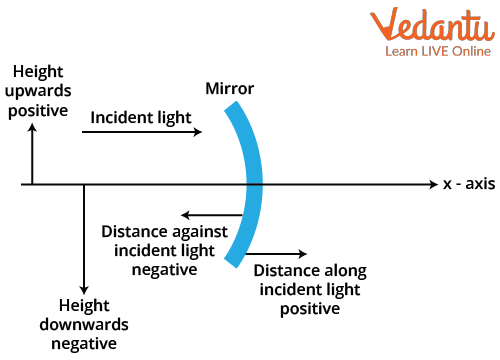Portraying the Sign Convention

As you can see, the height of the object upwards is taken as positive in front of a mirror and is taken negatively if it is downwards. This is what the signs of convention look like. Let us discuss what is the power of the lens, what is sign convention and its rules in detail.

## Power of Lens

The power of a lens as described in ray optics is its capability to curve the light. The larger the power of a lens, the larger its capability to refract light that passes through it.  The power of the lens can be computed by the following formula:

\$p=\dfrac{1}{f}\$

where f= the focal length of the lens.

Lenses bend the light that moves across them. The orientation and amount of the bending of light depend on the curvature of the lens, the lens material, and the material in which the lens is submerged (we assume that the lens is in the air). If both sides of the lens curve exteriorly, then it is called a converging lens. It will bend light from obscure objects within the direction of a single point, called the focal point.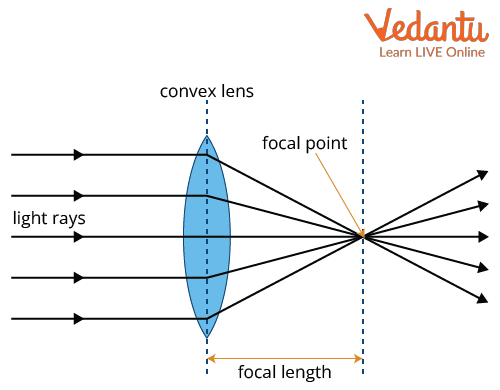Converging Lens

If both sides of the lens curve inward, then it is called a diverging lens. The light from obscure objects will bend outwards. Since the light is not being bent toward a single point, as it was in the case of converging lenses, it means that the focal point is on the same side of the lens.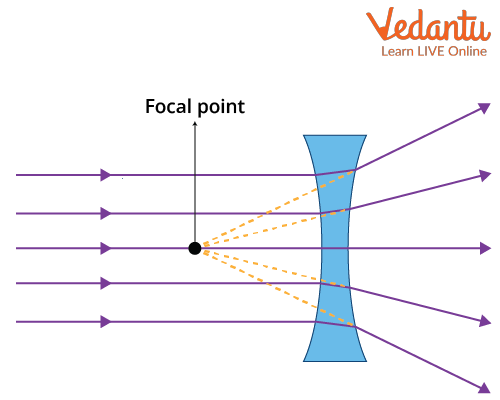Diverging Lens

The interspace between the lens and the focal point is termed as the focal length. For converging lenses, the focal length is always positive, but in the case of diverging lenses, the focal length becomes negative. However, these arrangements are inconsistent and can be changed.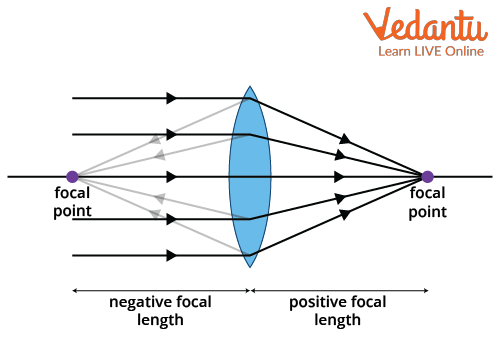Focal Length

The distance on the left of the mirror is against the incident rays and is thus taken as negative whereas the distance along with the lens beside the mirror is taken as positive as it is in the direction of the incident rays. Let us learn about the sign convention in lenses like the sign convention for spherical lenses and convex and concave lens sign conventions in detail.

## Rules of Sign Convention in Lens

### Spherical Lens

A spherical lens is also known as a singlet which is an optical lens with a curved surface that causes light rays to converge or diverge. Spherical lenses are extensively used in many parts as they extrapolate images onto the sensor without affecting their aspect ratios.

According to the spherical lens, the sign convention is as follows:

• The object should always be to the left of the lens.

• All the distances are calculated from the ocular centre of the mirror.

• Distances that are calculated in the orientation of the incident ray are positive and the distances calculated  in the direction opposite to the incident rays are negative.

• Sign convention can be countermanded and will still give accurate results.

This is the sign convention for spherical lenses.

### Convex Lens

There are numerous interesting situations observed when light from an object is passing through the converging and diverging lenses. Let us discuss that. Considering a situation where we have an object kept on one side of a converging lens, the image object will be beyond the lens from the actual object and will be inverted. In the same way with the focal point, if the image is on the opposite side of the lens from the object, the distance from the lens to the image will be positive.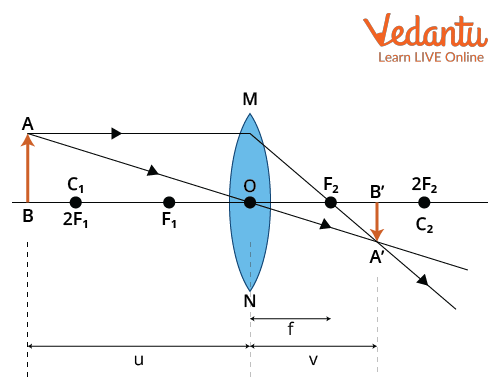Image Formation in Converging Lens

If the object is closer to the lens and if the object distance is smaller than the focal length, let us discuss what will be the focal length.Sign Convention of Convex Lens when the Object is between the Focal Point and the Centre of the Lens

In this situation, the image will be on the same side of the lens from the object and will be upstanding. The image will be larger than the object, which means that the distance of the image will be negative. This is the convex lens sign convention.

### Concave Lens

If the light passes through a diverging lens then what will be the situation? Let us see. In a concave lens, when an object is placed at a finite distance from the lens, the image of the object is formed in front of the object with a smaller size which is virtual and erect. The image will always be on the same side of the lens as the object, upstanding and relatively smaller than the object. As a result, the image distance for the diverging lens is negative. This is the concave lens sign convention.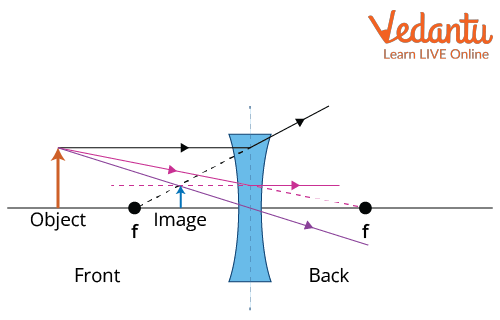Image Formation in Diverging Lens

## Magnification

This refers to the conversion of the size of the object from large to small and vice versa. It plays an important role in image formation in lenses. If the magnification is greater than one, the image is larger than the object, but if the magnification is smaller than one, the image is smaller than the object. For example, if the magnification is one-half, then the image seems to be half the size of the object.

The magnification of any lens is defined as the ratio of the height of the image to the height of the object. Mathematically, if the height of the image is represented by hi and the height of the object is represented by ho then the magnification m can be written as:

\$m=\dfrac{h_i}{h_o}\$

## Magnification Sign Convention

The sign of magnification also plays a vital role in determining the direction of the image. If the sign is positive, the image will become upstanding. If the sign is negative, then the image will be upturned. Moreover, the amount by which an object will be magnified interchanges depending on its distance from the focal point.

### Lens Sign Convention Table

 Quantity Condition Sign Focal Length, f Convex LensConcave Lens Positive (+)Negative (-) Object Distance Always Negative (-) Image Distance Image RealImage Virtual Positive (+)Negative (-) Magnification Image UprightImage Upturn Positive (+)Negative(-)

## Conclusion

Sign convention in lenses plays an important role in the image quality and formation. This article has given all the necessary details about this topic. To know about  the orientation of the image, the sign convention is needed.  Also, the article gives us an idea about the working principle of different lenses and their properties. When the light passes through the convex and concave lens, the behaviour is different for these two.

The convex lens behaves like a converging lens because it converges the light and produces the image while the concave lens behaves like a diverging lens as it diverges the incoming light to produce images. We also learnt about the lens sign convention table and had a thorough understanding of the magnification sign convention.

## FAQs on Sign Convention in Lens - Power, Rule, and Magnification for JEE

1. Can a convex lens form an image of the same size as that of the object?

Convex Lens forms an image the same size as that of the object when an object is placed at a distance which is double its focal length that is u=2f. Here, u is the object distance from the optical centre of the lens. The image formed by the convex lens will be the same size as the size of the object. The image formation depends on the position of the object from the focal length of the lens.

2. Write a few applications for a mirror and a lens.

The applications of a lens are listed below:

• Lenses are used in microscopes.

• Wearing glasses or spectacles.

• Telescopes are made up of lenses.

• Lens is used in magnifying glasses.

• Attenuate light.

The applications of a mirror are listed below:

• Mirrors are used in automobile headlights.

• Solar appliances also use mirrors.

• Our houses have mirrors which we use daily.

• Different mirrors are used in photography.

• Energy and affirmation techniques.

• Decorations also use mirrors as they reflect light and create an illusion of open space by doubling whatever is present in the room.